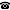# 2014 seminar talk: Copies of structures and ultrahomogeneous digraphs

Talk held by Miloš S. Kurilić (University of Novi Sad, Serbia) at the KGRC seminar on 2014-06-05.

### Abstract

The equality, isomorphism and equimorphism are natural relations between relational structures expressing different degrees of their similarity. In addition, if $\mathbb P(\mathbb X)$ denotes the poset of domains of substructures of a structure $\mathbb X$ isomorphic to $\mathbb X$, then the conditions $\mathbb P(\mathbb X)=\mathbb P(\mathbb Y)$, $\mathbb P(\mathbb X)\cong\mathbb P(\mathbb Y)$, $\mathop{\rm sq}\nolimits\mathbb P(\mathbb X)\cong\mathop{\rm sq}\nolimits\mathbb P(\mathbb Y)$ and $\mathop{\rm ro}\nolimits\mathop{\rm sq}\nolimits\mathbb P(\mathbb X) \cong \mathop{\rm ro}\nolimits\mathop{\rm sq}\nolimits\mathbb P(\mathbb Y)$ extend the list of such similarities; the last one is the roughest and equivalent to the forcing equivalence of the posets $\mathbb P(\mathbb X)$ and $\mathbb P(\mathbb Y)$. We will review some results concerning the hierarchy between these similarities and the corresponding classifications of structures. Also we will present some recent results concerning the structures with strongly connected components and a classification of ultrahomogeneous binary structures.

Kurt Gödel Research Center for Mathematical Logic. Währinger Straße 25, 1090 Wien, Austria.+43-1-4277-50501. Last updated: 2010-12-16, 04:37.